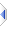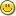# Floats Are Not Exact

While all integer values (up to a certain limit, around 1015 on 32-bit and 1019 on 64-bit) are always exact, the same cannot be said for floating point numbers.

As mentioned in Number Bases, computers hold numbers in binary, or base 2. And just as our familiar decimal system cannot hold 1/3 (one third) exactly, neither can base 2, which also cannot hold 1/5 (one fifth) exactly. It may only be out by the tiniest of amounts, less than, say, the width of an atom (pun intended), but it simply cannot be exact. The principle of "close enough" applies.

0.1 in decimal is held in binary as 0.0001100110011001100110011001100110011001100110011... i.e. 0/2+0/4+ 0/8+1/16+ 1/32+0/64+ 0/128+1/256+ 1/512+0/1024, etc. It gets pretty close, but would never manage to be exact, no matter how many digits you used. In fact the only fractions that can be held exactly are a half, quarter, eighth, etc, and any value that can be composed as an exact sum of such fractions.

A related but different concept is precision: starting with a value of 1e300, you can try to add 1 (or for that matter 1e250) to it as many times as you like; it will never make the slightest dent, not even if you leave it running for millenia. (Just something you should know.) Obviously if you multiply the 1 (or the 1e250) by something before the addition, that’s different. The actual precision available is about 15 decimal digits on 32 bit and about 19 decimal digits on 64 bit, and accordingly if the ratio between two numbers is too extreme, we find ourselves in one of those too small to make a dent situations. If we were only keeping numbers to two decimal places, then the most accurate answer for 3.00 plus 0.001 is still 3.00, and something similar happens when we are limited to 15 or 19 significant decimal digits. You get a very different answer from ((15+(1e-30))-15) [ie 0] to ((15-15)+(1e-30)) [ie 1e-30], and likewise 1e300+1-1e300 yields 0 whereas 1e300-1e300+1 yields 1.

I also rather like the following analogy. On 32-bit you can use atoms to store integer values up to 9,007,199,254,740,992 (ie 2^53), but like a bookshelf without any end stops, if you need an extra bit on the left, then one must fall off the right. In fact, between 9,007,199,254,740,992 and 18,014,398,509,481,984 you can only store even numbers, and between 18,014,398,509,481,984 and 36,028,797,018,963,968, you can only store numbers divisible by 4, and so on, until by the time you get to 1e300 the "smallest increment" is around 1e285. On 64-bit you can use atoms to store integer values up to 18,446,744,073,709,551,616 (ie 2^64). Should you encounter a declaration such as atom limit = power(2,iff(machine_bits()=32?53:64) then you now know it defines the largest exact integer (with no prior gaps) that can be held in an IEEE 754 [extended precision, ie 64 or 80-bit] float, above which you cannot be sure that your result is not rounded to the nearest 2/4/8/16/etc.

Of course such approximations occur in our familiar decimal system, and in the real world are more prevalent than not. You and I might say 31mm, an engineer 31.147mm, and a physicist 31.147436892mm, before adding something completely incomprehensible about lengths being composed of an infinite series of quantum probability functions being distorted by gravitational waves, or something like that. It can be annoying that you need to take extra care when dealing with pennies and cents, but it is inevitable for any hardware that can also cope with the kind of numbers needed for astrophysics.

These features of the physical hardware are common to all programming languages, at least when using the native machine floating point hardware as opposed to a specialised fraction or decimal library component such as bigatom, which operates digit-by-digit much like you or I would perform calculations using pencil and paper, and therefore is considerably slower, or better yet mpfr, which is highly optimised and certainly much faster than I ever imagined was possible.

In particular, comparing floating point numbers for equality is almost always the wrong thing to do.

Instead of `a=10.0` use `a>9.99 and a<10.01`, and instead of `a=b` use `abs(a-b)<1e-6`, or similar.

Alternatively it might be reasonable to design an accounting system that internally works in whole pennies/cents, and multiplies by 0.01 (or whatever) just before the display. Speaking of accounting systems, and more than wildly exagerrating the danger, imagine that some odd 5p is actually being stored as 0.0497 - it would take a mere 17 additions, such that remainder(sum,0.05) is less than 0.0450, before a penny discrepancy suddenly materialised out of nowhere. Of course in a real system, it would take more than a million million (1012) additions for such an error to arise, though that number tumbles once multiplication gets involved. Perhaps converting fractions to whole pennies and back on a regular basis would help, the point being made here is that there is always a way to minimise the impact of the tiny imperfections inherent in IEEE 754 floating points.

It is for all these reasons that Phix does not and never will permit floating point for loops; they simply do not work as expected, besides it is trivial to use an integer control loop variable and a manually maintained floating point value to achieve the desired results, without occasionally iterating once more or once less than was originally intended. Of course the real problem with floating point for loops is the overwhelming temptation to embed equality comparisons in them, which as said above in bold italics, is usually wrong.

See wikipedia or python docs if for whatever reason you think I might be making all this stuff up.Note that floating point inaccuracies tend to be more evident on 64-bit. This is because Phix exclusively uses the standard FPU registers (st0..st7) which are fundamentally 80-bit; while 32-bit Phix stores 64-bit floats, 64-bit stores 80-bit floats. Imagine that instead of 64/80 binary bits we had 2/4 decimal places, and that some calculation led to a result of 1.9994 - if we store to 4dp it stays 1.9994, whereas if we store to 2dp then (as if by magic) it becomes 2.0. There is a thin veneer of rounding modes that hide a multitude of sins in 32-bit, that are simply not available in 64-bit, at least not in the 1:1 way that most of the code has been translated. More specifically, on 32-bit we store 80-bit FPU registers in 64-bit floats, forcing a little bit of rounding, that simply does not happen when we store an 80-bit FPU register in an 80-bit float. These problems would quite probably evaporate if it[64-bit] used SSE/xmm0..7 [128-bit regs], which is probably not all that technically difficult, but finding the time and motivation is a different matter.